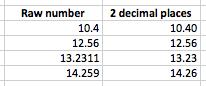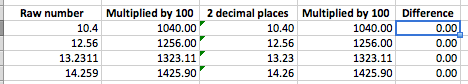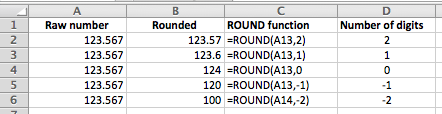# Rounding numbers in Excel

Rounding in Excel refers to reducing the number of digits in a number to make it easier to work with. A common example is rounding a price to two decimal places. Rounding errors can cause havoc with your spreadsheets without you even realising it. A common mistake occurs when you change the display format of a number to show fewer digits after the decimal point and assume that the number has been rounded for use in other calculations. This lesson explains how rounding in Excel works, and shows you how to use the different rounding functions available in Excel.

## Rounding versus Formatting in Excel

As I've already mentioned, a very common mistake people make when creating Excel spreadsheets is to assume that using the formatting options in Excel to change how numbers display also causes those numbers to be rounding. Even seasoned Excel professionals get caught out by this one, and end up scratching their head while they try to figure out why their calculations don't work out as expected. To see what I mean, check out these examples.

• Imagine you have a spreadsheet with the following numbers which you format to only show two decimal places:• Note how the third and fourth numbers look different when rounded down to two decimal places. The reality is that both columns are the same - they just look different because of the formatting. Here's what happen when you multiple the numbers in each column by 100:
• In the next example, there is no difference between the numbers in the second and fourth columns - even though the numbers in column three look different, the numbers being stored by Excel are the same. If you want Excel to see them differently, you will need to use one of the Rounding functions to round the numbers to the number of digits or decimal points you want to work with.## The ROUND function

The simplest way to round numbers is to use the ROUND function:

=ROUND(number,num_digits)

• The values in this function are as follows:
• number is the number you want to round. This can be a number, a reference to a cell with a number in it, or the result of another calculation inside a cell.
• num_digits is the number of digits the number should be rounded to.
• You can use num_digits in several ways depending on the result you want:
• If num_digits is greater than zero, the ROUND function will round your number to that many digits after the decimal point.
• If num_digits is zero, the ROUND function will round your number to the nearest integer (i.e. the nearest whole number)
• If num_digits is less than zero, the ROUND function will round the digits to the left.
• The following example shows how ROUND can be used to round a number to two decimal points (column C in this example shows the formula used in column B):• This example shows ROUND with a range of values for num_digits ranging from -2 to 2. Note how the negative numbers round the values to the nearest 1 (if set to 0), the nearest 10 (if set to -1), and the nearest hundred (if set to -2):• You could also use the ROUND function inside another formula, as shown in this example. Note how the ROUND function is used to round the values in column A before completing the calculation, which is to multiply the rounded number by 10.## Our Comment Policy.

Add a comment to this lesson

### Thank you!!

I am learning so much from this site. Thanks for posting all these essential lessons.

### Somethin not right to me

You is goin on bout this FOUND function at the end there but you is meanin ROUND right, eva sort it out man or make it like clearer you get me?

### Fixed

Phillip - thanks for pointing that out. I've fixed it now.

David